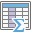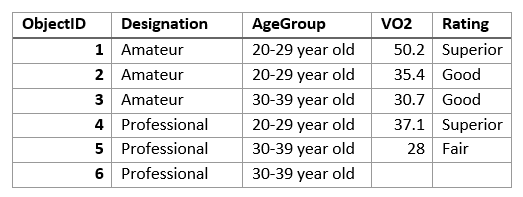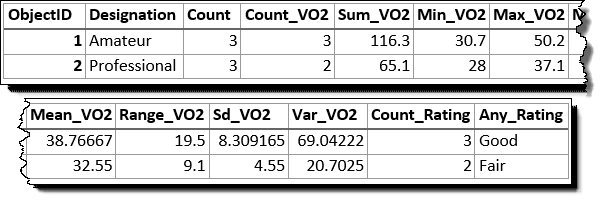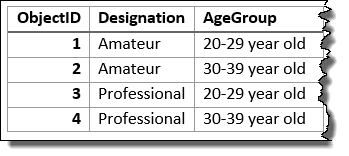Skip To Content

# Summarize AttributesThe Summarize Attributes tool summarizes like field values to generate a summary table. The resulting layer displays the count of features that have been summarized, as well as any additional statistics that have been specified.

## Analysis using GeoAnalytics Tools

Analysis using GeoAnalytics Tools is completed using distributed processing across multiple ArcGIS GeoAnalytics Server machines and cores. GeoAnalytics Tools and standard feature analysis tools in Portal for ArcGIS have different parameters and capabilities. To learn more about the differences between the tools, see Feature analysis tool differences.

## Examples

Tornadoes and hurricanes are some of the most violent types of storms that occur in the United States. You want to know property damage and financial loss for tornadoes and hurricanes to compare how their impact differs. You have access to tornado and hurricane data across the United States in a single dataset, and you want to summarize all the information to see a summary of values for all hurricanes and a summary of values for all tornadoes. You can summarize your data using the type of storm to determine the statistics for each storm type.

## Usage notes

Summarize Attributes is a tabular analysis tool, not spatial.

The most basic aggregations will calculate a count of the number of features that have been summarized. Statistics (count, sum, minimum, maximum, range, mean, standard deviation, and variance) can also be calculated on numerical fields, and statistics (count and any) can be calculated on string fields. The statistics will be calculated on each group separately.

You can specify one or more fields to summarize by or summarize all features. When you summarize by fields, statistics are calculated for each unique combination of attribute values.

The processing spatial reference and spatial reference of your input data will not affect your results.

If Use current map extent is checked, only the features that are visible within the current map extent will be analyzed. If unchecked, all input features in the input layer will be analyzed, even if they are outside the current map extent.

## Limitations

Inputs may be a tabular layer or a layer with geometry (points, lines, or areas).

You can apply this tool to spatial data, and you will get a tabular result. You can join your results to spatial data using Join Features.

## How Summarize Attributes works

### Equations

Variance is calculated using the following equation:

Standard deviation is calculated as the square root of the variance.

### Calculations

Input layers are summarized into groups with matching field values. The results are tabular, so they cannot be visualized on your map.

The tables below illustrate the statistical calculations of a layer that is summarized using like values of fields. The VO2 field was used to calculate the numeric statistics (Count,Sum, Minimum, Maximum, Range, Mean, Standard Deviation, and Variance) for the layer. The Rating field was used to calculate the string statistics (Count and Any) for the layer.Input layer to be summarized.

The table above was summarized on the field Designation, and the VO2 field was used to calculate the numeric statistics (Count,Sum, Minimum, Maximum, Range, Mean, Standard Deviation, and Variance) for the layer. The Rating field was used to calculate the string statistics (Count and Any) for the layer. This results is a table with two features, representing the distinct values of Designation.The input layer that has been summarized using the field Designation.

The following table represents what the first few fields look like when the layer is summarized using the Designation and Age Group fields. Statistics are calculated using the same methods as the previous example.The input layer that has been summarized using the fields Designation and Age Group.

The count statistic (for strings and numeric fields) counts the number of nonnull values. The count of the following values equals 5: [0, 1, 10, 5, null, 6] = 5. The count of this set of values equals 3: [Primary, Primary, Secondary, null] = 3.

## Similar tools

Use Summarize Attributes to summarize features with like values. Other tools may be useful in solving similar but slightly different problems.

### Map Viewer analysis tools

If you are trying to summarize points, lines, or areas using different spatial relationships, use the GeoAnalytics Tools Join Features.

### ArcGIS Desktop analysis tools

The GeoAnalytics Tools Summarize Attributes is available in ArcGIS Pro.

Summarize Attributes performs the functions of the Summary Statistics tool.